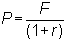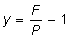﻿ 2.2 Bond Prices: Single-Period Caseoffice (412) 9679367
fax (412) 967-5958
toll-free 1 (800) 214-3480

## 2.2 Bond Prices: Single-Period Case

 T

he present value of a bond can be derived, given interest rates, by applying the principle of discounting and assuming that no arbitrage opportunity exists. We argue here that this value must equal the price of the bond. Otherwise, there is an arbitrage opportunity.

Let us apply these arguments first to a zero-coupon, or pure-discount, bond. Suppose the bond pays its face value, F, in one period's time. Let the bond's price equal P and suppose that r is the one-period interest rate at which investors can borrow or lend in the market. If there is no arbitrage, then the price of the bond must satisfy:

P(1+r) = F

orTo see why, suppose P(1 + r) < C. Now you should borrow P at interest rate r and buy the bond. In the next period, you will owe P(1 + r) but will get F > P(1 + r) and will have made a pure arbitrage profit. Therefore, at least P(1 + r) > F. However, if P(1 + r) > F, you should sell the bond and invest the proceeds at interest rate r. In the next period, you will owe F but your investment will be worth P(1 + r) > F. This implies P(1 + r) < F. Taken together,Example 1: Determining the Arbitrage-Free Price of a Bond

If the face value of a zero-coupon bond equals \$100, and the interest rate is 5%, then the arbitrage-free price of the bond is:Alternatively, suppose you observe that the price of a one-period bond is P. Now, you can use the formula to determine the interest rate implicit in the price. This interest rate is the return (or yield) you would get if you bought the bond today at price P and held it to maturity.

In one period, the price of the bond must equal its face value, F. To determine your return, you have to ask: At what interest rate would I have to invest P in order to get F in one period ?

The answer is the number y, such that P(1 + y) = F. If you rearrange this, the yield iswhich simplifies toOf course, because the one-period borrowing and lending rate is r, the yield must equal r, otherwise there is an arbitrage opportunity. If the yield is y > r, then this can immediately be exploited by borrowing at r and buying the discount bond. If y < r, you should sell the discount bond and lend the proceeds at rate r.

Example II: Determining the Arbitrage-Free Price of a Bond

If the face value of a bond equals \$100 and the price is \$95.24, then the yield from this investment is approximately (rounding to two decimal places):In both of the above examples, it is straightforward to construct the arbitrage-free, pure discount bond price given the one-year interest rate, r.

The next topic, Arbitrage-Free Prices: Multi-Period Case, extends the above analysis.